﻿ Factor Analysis as a Tool for Survey AnalysisPublications are Open
Access in this journal
Article Versions
Export Article
• Normal Style
• MLA Style
• APA Style
• Chicago Style
Research Article
Open Access Peer-reviewed

Factor Analysis as a Tool for Survey Analysis

Noora Shrestha
American Journal of Applied Mathematics and Statistics. 2021, 9(1), 4-11. DOI: 10.12691/ajams-9-1-2
Received December 10, 2020; Revised January 11, 2021; Accepted January 20, 2021

Abstract

Factor analysis is particularly suitable to extract few factors from the large number of related variables to a more manageable number, prior to using them in other analysis such as multiple regression or multivariate analysis of variance. It can be beneficial in developing of a questionnaire. Sometimes adding more statements in the questionnaire fail to give clear understanding of the variables. With the help of factor analysis, irrelevant questions can be removed from the final questionnaire. This study proposed a factor analysis to identify the factors underlying the variables of a questionnaire to measure tourist satisfaction. In this study, Kaiser-Meyer-Olkin measure of sampling adequacy and Bartlett’s test of Sphericity are used to assess the factorability of the data. Determinant score is calculated to examine the multicollinearity among the variables. To determine the number of factors to be extracted, Kaiser’s Criterion and Scree test are examined. Varimax orthogonal factor rotation method is applied to minimize the number of variables that have high loadings on each factor. The internal consistency is confirmed by calculating Cronbach’s alpha and composite reliability to test the instrument accuracy. The convergent validity is established when average variance extracted is greater than or equal to 0.5. The results have revealed that the factor analysis not only allows detecting irrelevant items but will also allow extracting the valuable factors from the data set of a questionnaire survey. The application of factor analysis for questionnaire evaluation provides very valuable inputs to the decision makers to focus on few important factors rather than a large number of parameters.

1. Introduction

Factor Analysis is a multivariate statistical technique applied to a single set of variables when the investigator is interested in determining which variables in the set form logical subsets that are relatively independent of one another 1. In other words, factor analysis is particularly useful to identify the factors underlying the variables by means of clubbing related variables in the same factor 2. In this paper, the main focus is given on the application of factor analysis to reduce huge number of inter-correlated measures to a few representative constructs or factors that can be used for subsequent analysis 3. The goal of the present work is to examine the application of factor analysis of a questionnaire item to measure tourist satisfaction. Therefore, in order to identify the factors, it is necessary to understand the concept and steps to apply factor analysis for the questionnaire survey.

Factor analysis is based on the assumption that all variables correlate to some degree. The variables should be measured at least at the ordinal level. The sample size for factor analysis should be larger but the more acceptable range would be a ten-to-one ratio 3, 4. There are two main approaches to factor analysis: exploratory factor analysis (EFA) and confirmatory factor analysis (CFA). Exploratory factor analysis is used for checking dimensionality and often used in the early stages of research to gather information about the interrelationships among a set of variables 5. On the other hand, the confirmatory factor analysis is a more complex and sophisticated set of techniques used in the research process to test specific hypotheses or theories concerning the structure underlying a set of variables 6, 7.

Several studies examined and discussed the application of factor analysis to reduce the large set of data and to identify the factors extracted from the analysis 8, 9, 10, 11. In tourism business, the satisfaction of tourists can be measured by the large number of parameters. The factor analysis may cluster these variables into different factors where each factor measure some dimension of tourist satisfaction. Factors are so designed that the variables contained in it are linked with each other in some way. The significant factors are extracted to explain the maximum variability of the group under study. The application of factor analysis provides very valuable inputs to the decision makers and policy makers to focus on few factors rather than a large number of parameters. People related to the tourism business is interested to know as to what makes their customer or tourists to choose a particular destination. There may be boundless concerns on which the opinion of the tourists can be taken. Several issues like local food, weather condition, culture, nature, recreation activities, photography, travel video making, transportation, medical treatment, water supply, safety, communication, trekking, mountaineering, environment, natural resources, cost of accommodation, transportation, etc. may be explored by taking the responses from the tourists survey and from the literature review 12. By using the factor analysis, the large number of variables may be clubbed in different components like component one, component two, etc. Instead of concentrating on many issues, the researcher or policy maker can make a strategy to optimize these components for the growth of tourism business.

The contribution of this paper is twofold related to the advantages of factor analysis. First, factor analysis can be applied to developing of a questionnaire. On doing analysis, irrelevant questions can be removed from the final questionnaire. It helps in categorizing the questions into different parameters in the questionnaire. Second, factor analysis can be used to simplify data, such as decreasing the number of variables in regression models. This study also encourages researchers to consider the step-by-step process to identify factors using factor analysis. Sometimes adding more statements or items in the questionnaire fail to give clear understanding of the variables. Using factor analysis, few factors are extracted form the large number of related variables to a more manageable number, prior to using them in other analysis such as multiple regression or multivariate analysis of variance 7, 13. Hence, instead of examining all the parameters, few extracted factors can be studied which in turn explain the variations of the group characteristics. Therefore, the present study discusses on the factor analysis of a questionnaire to measure tourist satisfaction. In the present work, data collected from the tourist satisfaction survey is used as an example for the factor analysis.

2. Method

2.1. Instrument Reliability and Validity

The structured questionnaire was designed to collect primary data. The data were collected from the international tourists travelled various places of Nepal in 2019. The tourists older than 25 years of age who had been in Nepal for over a week and had experienced the travelling were included in this study. The pilot study was carried out among 15 tourists, who were not included in the sample, to identify the possible errors of a questionnaire so as to improve the reliability (Cronbach’s alpha > 0.7) of the questionnaire. The questionnaire consists of questions and statements related to the independent and dependent variables, which were developed on the basis of literature review. Each statement was rated on a five-point (1 to 5) Likert scale, with high score 5 indicating strongly agree with that statement. The statements were written to reflect the hospitality, destination attractions, and relaxation. The data were gathered from the 1st week of November 2019 to last week of December 2019. Due to outbreak of 2019 novel coronavirus, the data collection process was affected and hence convenience sampling method was used to select a respondent. Total 220 questionnaires were distributed among the tourists but only 200 respondents provided their reactions to the statements with a response rate 91%. All the statistical analysis has performed using IBM SPSS version 23.

2.1.1. Cronbach’s Alpha

The reliability of a questionnaire is examined with Cronbach’s alpha. It provides a simple way to measure whether or not a score is reliable. It is used under the assumption that there are multiple items measuring the same underlying construct; such as in tourist satisfaction survey, there are few questions all asking different things, but when combined, could be said to measure overall satisfaction. Cronbach’s alpha is a measure of internal consistency. It is also considered to be a measure of scale reliability and can be expressed as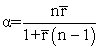where, n represents the number of items, and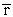is the mean correlation between the items. Cronbach’s alpha ranges between 0 and 1. In general, Cronbach’s alpha value more than 0.7 is considered as acceptable. A high level of alpha shows the items in the test are highly correlated 14.

2.1.2. Average Variance Extracted (AVE) and Composite Reliability (CR)

The average variance extracted and the composite reliability coefficients are related to the quality of a measure. AVE is a measure of the amount of variance that is taken by a construct in relation to the amount of variance due to measurement error 15. To be specific, AVE is a measure to assess convergent validity.

Convergent validity is used to measure the level of correlation of multiple indicators of the same construct that are in agreement. The factor loading of the items, composite reliability and the average variance extracted have to be calculated to determine convergent validity 16. The value of AVE and CR ranges from 0 to 1, where a higher value indicates higher reliability level. AVE is more than or equal to 0.5 confirms the convergent validity. The average variance extracted is the sum of squared loadings divided by the number of items and is given by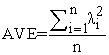Composite reliability is a measure of internal consistency in scale items 17. According to Fornell and Larcker (1981), composite reliability is an indicator of the shared variance among the observed variables used as an indicator of a latent construct. CR for each construct can be obtained by summing of squares of completely standardized factor loadings divided by this sum plus total of variance of the error term for ith indicators. CR can be calculated as: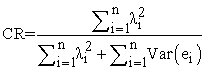Here, n is the number of the items, λi the factor loading of item i, and Var (ei) the variance of the error of the item i, The values of composite reliability between 0.6 to 0.7 are acceptable while in more advanced phase the value have to be higher than 0.7. According to Fornell and Larcker (1981), if AVE is less than 0.5, but composite reliability is higher than 0.6, the convergent validity of the construct is still adequate.

2.2. Factor Analysis

This study employs exploratory factor analysis to examine the data set to identify complicated interrelationships among items and group items that are part of integrated concepts. Due to explorative nature of factor analysis, it does not differentiate between independent and dependent variables. Factor analysis clusters similar variables into the same factor to identify underlying variables and it only uses the data correlation matrix. In this study, factor analysis with principal components extraction used to examine whether the statements represent identifiable factors related to tourist satisfaction. The principal component analysis (PCA) signifies to the statistical process used to underline variation for which principal data components are calculated and bring out strong patterns in the dataset 6, 9.

Factor Model with ‘m’ Common Factors

Let X = (X1, X2, ....Xp)’is a random vector with mean vector μ and covariance matrix Σ. The factor analysis model assumes that X = μ + λ F + ε, where, λ = { λ jk}pxm denotes the matrix of factor loadings; λ jk is the loading of the jth variable on the kth common factor, F= (F1,F2,....Fm)’ denotes the vector of latent factor scores; Fk is the score on the kth common factor and ε = (ε1, ε2,....εp)’ denotes the vector of latent error terms; εj is the jth specific factor.

2.2.1. Steps Involved in Factor Analysis

There are three major steps for factor analysis: a) assessment of the suitability of the data, b) factor extraction, and c) factor rotation and interpretation. They are described as:

2.2.1.1. Assessment of the Suitability of the data

To determine the suitability of the data set for factor analysis, sample size and strength of the relationship among the items have to be considered 1, 18. Generally, a larger sample is recommended for factor analysis i.e. ten cases for each item. Nevertheless, a smaller sample size can also be sufficient if solutions have several high loading marker variables < 0.80 18. To determine the strength of the relationship among the items, there must be evidence of the coefficient of correlation > 0.3 in the correlation matrix. The existence of multicollinearity in the data is a type of disturbance that alters the result of the analysis. It is a state of great inter-correlations among the independent variables. Multicollinearity makes some of the significant variables in a research study to be statistically insignificant and then the statistical inferences made about the data may not be trustworthy 19, 20. Hence, the presence of multicollinearity among the variables is examined with the determinant score.

Determinant Score

The value of the determinant is an important test for multicollinearity or singularity. The determinant score of the correlation matrix should be > 0.00001 which specifies that there is an absence of multicollinearity. If the determinant value is < 0.00001, it would be important to attempt to identify pairs of variables where correlation coefficient r > 0.8 and consider eliminating them from the analysis. A lower score might indicate that groups of three or more questions/statements have high inter-correlations, so the threshold for item elimination should be reduced until this condition is satisfied. If correlation is singular, the determinant |R| =0 20, 21.

There are two statistical measures to assess the factorability of the data: Kaiser-Meyer-Olkin (KMO) measure of sampling adequacy and Bartlett’s test of Sphericity.

Kaiser-Meyer-Olkin (KMO) Measure of Sampling Adequacy

KMO test is a measure that has been intended to measure the suitability of data for factor analysis. In other words, it tests the adequacy of the sample size. The test measures sampling adequacy for each variable in the model and for the complete model. The KMO measure of sampling adequacy is given by the formula: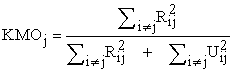where, Rij is the correlation matrix and Uij is the partial covariance matrix. KMO value varies from 0 to 1. The KMO values between 0.8 to 1.0 indicate the sampling is adequate. KMO values between 0.7 to 0.79 are middling and values between 0.6 to 0.69 are mediocre. KMO values less than 0.6 indicate the sampling is not adequate and the remedial action should be taken. If the value is less than 0.5, the results of the factor analysis undoubtedly won’t be very suitable for the analysis of the data. If the sample size is < 300 the average communality of the retained items has to be tested. An average value > 0.6 is acceptable for sample size < 100, an average value between 0.5 and 0.6 is acceptable for sample sizes between 100 and 200 1, 22, 23, 24.

Bartlett’s Test of Sphericity

Bartlett’s Test of Sphericity tests the null hypothesis, H0: The variables are orthogonal i.e. The original correlation matrix is an identity matrix indicating that the variables are unrelated and therefore unsuitable for structure detection. The alternative hypothesis, H1: The variables are not orthogonal i.e. they are correlated enough to where the correlation matrix diverges significantly from the identity matrix. The significant value < 0.05 indicates that a factor analysis may be worthwhile for the data set.

In order to measure the overall relation between the variables the determinant of the correlation matrix |R| is calculated. Under H0, |R| =1; if the variables are highly correlate, then |R| ≈ 0. The Bartlett’s test of Sphericity is given by: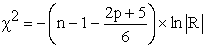where, p= number of variables, n= total sample size and R= correlation matrix 22, 24.

2.2.1.2. Factor Extraction

Factor extraction encompasses determining the least number of factors that can be used to best represent the interrelationships among the set of variables. There are many approaches to extract the number of underlying factors. For obtaining factor solutions, principal component analysis and common factor analysis can be used. This study has used principal component analysis (PCA) because the purpose of the study is to analyze the data in order to obtain the minimum number of factors required to represent the available data set.

To Determine the Number of Factors to be Extracted

In this study two techniques are used to assist in the decision concerning the number of factors to retain: Kaiser’s Criterion and Scree Test. The Kaiser’s criterion (Eigenvalue Criterion) and the Scree test can be used to determine the number of initial unrotated factors to be extracted. The eigenvalue is a ratio between the common variance and the specific variance explained by a specific factor extracted.

Kaiser’s (Eigenvalue) Criterion

The eigenvalue of a factor represents the amount of the total variance explained by that factor. In factor analysis, the remarkable factors having eigenvalue greater than one are retained. The logic underlying this rule is reasonable. An eigenvalue greater than one is considered to be significant, and it indicates that more common variance than unique variance is explained by that factor 7, 22, 23, 25. Measure and composite variables are separate classes of variables. Factors are latent constructs created as aggregates of measured variables and so should consist of more than a single measured variable. But eigenvalues, like all sample statistics, have some sampling error. Hence, it is very important for the researcher to exercise some judgment in using this strategy to determine the number of factors to extract or retain 26.

Scree Test

Cattell (1996) proposed a graphical test for determining the number of factors. A scree plot graphs eigenvalue magnitudes on the vertical access, with eigenvalue numbers constituting the horizontal axis. The eigenvalues are plotted as dots within the graph, and a line connects successive values. Factor extraction should be stopped at the point where there is an ‘elbow’ or leveling of the plot. This test is used to identify the optimum number of factors that can be extracted before the amount of unique variance begins to dominate the common variance structure 4, 27, 28.

2.2.1.3. Factor Rotation and Interpretation

Orthogonal Factor Model Assumptions

The orthogonal factor analysis model assumes the form X = μ + λ F + ε, and adds the assumptions that F~ (0, 1m), i.e. the latent factors have mean zero, unit variance, and are uncorrelated, Ε ~ (0, Ψ) where Ψ = diag(Ψ1, Ψ2, ... Ψp) with Ψi denoting the jth specific variance, and εj and Fk are independent of one another for all pairs, j, k.

Variance Explained by Common Factors

The portion of variance of the jth variable that is explained by the ‘m’ common factors is called the communality of the jth variable: σjj = hj2 + ψj, where, σjj is the variance of Xj (i.e. jth diagonal of Σ). Communality is the sum of squared loadings for Xj and given by hj2 = (λλ’)jj = λ j12 + λ j22 +......+ λ jm2 is the communality of Xj, and ψj is the specific variance (or uniqueness) of Xj 14, 24, 26.

3. Results and Discussions

In this section the results obtained with the statistical software SPSS are presented. In this study, the participants consisted of 200 tourists who had been travelling in Nepal during 2019. Majority (26.5%) of tourists belongs to the age group 40 to 44 years. Participants ranged in age from 25 to 55 years (mean age= 39.8 years, standard deviation = 7.94) and of the total sample n=108, 54% were male and n= 92, 46% were female. In addition, the respondents were from various parts of the world. The region wise distribution of tourists was Asian–SAARC (n=59, 29.5%), Asian-others (n=58, 29%), European (n=40, 20%), Americans (n=18, 9%), Oceania (n=17, 8.5%), and Other (n=8, 4%). There are various purposes of visiting Nepal. 29.5% (n=59) of the tourist visited Nepal with the purpose of holiday and pleasure. Similarly, n=50, 25% of the tourist came for adventure including trekking and mountaineering, n=30, 15% for volunteering and academic purposes, n=44, 22% for entertainment video and photography, n=17, 8.5% for other purposes. The average length of stay of respondent tourists was found to be 12 days. According to Nepal tourism statistics 2019, the average length of stay of international tourists in Nepal in 2018 dropped to 12.4 days from 12.6 days in 2017 30.

3.1. Steps Involved in Factor Analysis

This study has followed three major steps for factor analysis: a) assessment of the suitability of the data, b) factor extraction, and c) factor rotation and interpretation.

Step 1: Assessment of the Suitability of the Data

The utmost significant factor of international tourist’s satisfaction is hospitality such as home stay and local family, arts, crafts, and historic places, local souvenirs, and local food. Similarly, destination attraction plays a vital role in tourist satisfaction such as cultural activities, trekking, sightseeing, and safety during travel period. Most of the tourists visit different places for relaxation and experience different lifestyle. These factors may be associated with the satisfaction of tourists. To analyze the tourist satisfaction, Kaiser-Meyer-Olkin is used to measure the suitability of data for factor analysis. Similarly, Bartlett’s test of Sphericity, correlation matrix, and determinant score are computed to detect the appropriateness of the data set for functioning factor analysis 31.

In Table 1, the correlation matrix displays that there are sufficient correlations to justify the application of factor analysis. The correlation matrix shows that there are few items whose inter-correlations > 0.3 between the variables and it can be concluded that the hypothesized factor model appears to be suitable. The value for the determinant is an important test for multicollinearity. The determinant score of the correlation matrix is 0.038 > 0.00001 which indicates that there is an absence of multicollinearity.

Table 2 illustrates the value of KMO statistics is equal to 0.813 > 0.6 which indicates that sampling is adequate and the factor analysis is appropriate for the data. Bartlett’s test of Sphericity is used to test for the adequacy of the correlation matrix. The Bartlett’s test of Sphericity is highly significant at p < 0.001 which shows that the correlation matrix has significant correlations among at least some of the variables. Here, test value is 637.65 and an associated degree of significance is less than 0.0001. Hence, the hypothesis that the correlation matrix is an identity matrix is rejected. To be specific, the variables are not orthogonal. The significant value < 0.05 indicates that a factor analysis may be worthwhile for the data set.

Step 2: Factor Extraction

Kaiser’s criterion and Scree test are used to determine the number of initial unrotated factors to be extracted. The eigenvalues associated with each factor represent the variance explained by those specific linear components. The coefficient value less than 0.4 is suppressed that will suppress the presentation of any factor loadings with values less than 0.4 23.

Table 3 demonstrates the eigenvalues and total variance explained. The extraction method of factor analysis used in this study is principal component analysis. Before extraction, eleven linear components are identified within the data set. After extraction and rotation, there are three distinct linear components within the data set for the eigenvalue > 1. The three factors are extracted accounting for a combined 60.2% of the total variance. It is suggested that the proportion of the total variance explained by the retained factors should be at least 50%. The result shows that 60.2% common variance shared by eleven variables can be accounted by three factors. This is the reflection of KMO value, 0.813, which can be considered good and also indicates that factor analysis is useful for the variables. This initial solution suggests that the final solution will extract not more than three factors. The first component has explained 22% of the total variance with eigenvalue 4.01. The second component has explained 20.9% variance with eigenvalue 1.54. The third component has explained 17.34% variance with eigenvalue 1.08.

In Figure 1, for Scree test, a graph is plotted with eigenvalues on the y-axis against the eleven component numbers in their order of extraction on the x-axis. The initial factors extracted are large factors with higher eigenvalues followed by smaller factors. The scree plot is used to determine the number of factors to retain. Here, the scree plot shows that there are three factors for which the eigenvalue is greater than one and account for most of the total variability in data. The other factors account for a very small proportion of the variability and considered as not so much important.

Step 3: Factor Rotation and Interpretation

The present study has executed the extraction method based on principal component analysis and the orthogonal rotation method based on varimax with Kaiser normalization.

Table 4 exhibits factor loading, diagonal anti-image correlation and communality after extraction. The diagonal anti-image correlation stretches the knowledge of sampling adequacy of each and every item. The communalities reflect the common variance in the data structure after extraction of factors. Factor loading values communicates the relationship of each variable to the underlying factors. The variables with large loadings values > 0.40 indicate that they are representative of the factor.

• Figure 1. Scree Plot

The component 1 is labeled as ‘Hospitality’ which contains four items that strive for homestay, local food, local souvenirs, arts and craft, and have a correlation of 0.77, 0.70, 0.71, and 0.74, with component 1 respectively. The component hospitality explained 22% of the total variance with eigenvalue 4.01. This component contained four items but out of these items the arts, craft, and historic places tends to be strongly agreeing according to its mean score 4.23. The other three items such as strive for homestay, arts & historic place, local souvenirs, and local food have a tendency towards agree according to their mean score of the scale.

The second component entitled as ‘Destination Attraction’ explained 20.9% variance with eigenvalue 1.54. This component contained four items such as sightseeing, trekking, cultural activities, and safety. The variables sightseeing, trekking, cultural activities, and safety have correlation of 0.71, 0.72, 0.79, and 0.58 with component 2 respectively. The item cultural activities (mean = 4.25) tends to strongly agree but other items trekking, sightseeing, and safety tend to agree according to their mean score of scale.

The component 3 is marked as ‘Relaxation’. It contains three items namely stress relief, different lifestyle, new experience and which have a correlation of 0.52, 0.86, and 0.84 with component 3 respectively. The third component explained 17.34% variance with eigenvalue 1.08. The three items of the third component such as different lifestyle, new experience, and stress relief, tend to agree according to their mean score of scale.

In Table 4, the diagonal element of the anti-image correlation value gives the information of sampling adequacy of each and every item that must be > 0.5. The amount of variance in each variable that can be explained by the retained factor is represented by the communalities after extraction. The communalities suggest the common variance in the data set. The communality value corresponding to the first statement (Item_1) of the first component is 0.63. It means 63% of the variance associated with this statement is common. Similarly, 0.63%, 0.57%, 0.56%, 0.64%, 0.59%, 0.55%, 0.67%, 0.50%, 0.50%, 0.77%, and 0.72% of the common variance associated with statement first to eleventh respectively.

4. Reliability and Validity Test Results

The internal consistency is confirmed by calculating Cronbach’s alpha to test the instrument accuracy and reliability. The adequate threshold value for Cronbach’s alpha is that it should be > 0.7. In Table 5 the component hospitality, destination attraction, and relaxation have Cronbach’s alpha values 0.75, 0.74, and 0.71 respectively, which confirmed the reliability of the survey instrument. The Cronbach’s alpha coefficient for the factors with total scale reliability is 0.82 > 0.7. It shows that the variables exhibit a correlation with their component grouping and thus they are internally consistent.

The convergent validity is established when average variance extracted is ≥ 0.5. The AVE values corresponding to the components hospitality, destination attraction, and relaxation are 0.53, 0.50, and 0.56 respectively. According to Fornell and Larcker (1981), AVE ≥ 0.5 confirms the convergent validity and it can be seen that all the AVE values in Table 5 are greater or equal to 0.5. The composite reliability value for component 1, 2, and 3 are 0.82, 0.79, and 0.79 respectively. It evidences the internal consistency in scale items.

5. Conclusion

The goal of this study was to examine on the factor analysis of a questionnaire to identify main factors that measure tourist satisfaction. The likelihood to use factor analysis for the data set is explored with the threshold values of determinant score, Kaiser-Meyer-Olkin and Bartlett’s test of Sphericity. Based on the results of this study, it can be concluded that factor analysis is a promising approach to extract significant factors to explain the maximum variability of the group under study.

The hospitality, destination attraction, and relaxation are the major factors extracted using principal component analysis and varimax orthogonal factor rotation method to measure satisfaction of tourists. The application of factor analysis provides very valuable inputs to the decision makers and policy makers to focus only on the few manageable factors rather than a large number of parameters. The findings of the study cannot be generalized for the large population so advanced study can be done taking more sample size with probability sampling methods. Nevertheless, before making stronger decision on the tourist satisfaction factors to promote tourism of a country, further research is required to analyze in detail.

References

  Tabachnick, B.G. and Fidell, L.S., Using multivariate statistics (6th ed.), Pearson, 2013. In article  Verma, J. and Abdel-Salam, A., Testing statistical assumptions in research, John Willey & Sons Inc., 2019. In article View Article  Ho, R., Handbook of univariate and multivariate data analysis and interpretation with SPSS, Chapman & Hall/CRC, Boca Raton, 2006. In article View Article  Hair, J.F., Anderson, R.E., Tatham, R.L., and Black, W.C., Multivariate data analysis (5th ed.), N J: Prentice-Hall, Upper Saddle River, 1998. In article  Pituch, K. A. and Stevens, J., Applied multivariate statistics for the social sciences: Analyses with SAS and IBM’s SPSS (6th ed.), Taylor & Francis, New York, 2016. In article View Article  Hair, J. J., Black, W.C., Babin, B. J., Anderson, R. R., Tatham, R. L., Multivariate data analysis, Upper Saddle River, New Jersey, 2006. In article  Pallant, J., SPSS survival manual: a step by step guide to data analysis using SPSS, Open University Press/ Mc Graw-Hill, Maidenhead, 2010. In article  Cerny, C.A. and Kaiser, H.F, “A study of a measure of sampling adequacy for factor analytic correlation matrices,” Multivariate Behavioral Research, 12 (1). 43-47. 1977. In article View Article  PubMed  Dziuban, C.D. and Shirkey, E.C., “When is a correlation matrix appropriate for factor analysis?” Psychological Bulletin, 81, 358-361. 1974. In article View Article  Bartlett, M. S., “The effect of standardization on a Chi-square approximation in factor analysis,” Biometrika, 38(3/4), 337-344. 1951. In article View Article  MacCallum, R.C., Widaman, K. F., Zhang, S. and Hong, S. “Sample size in factor analysis,” Psychological Methods, 4(1), 84-99. 1999. In article View Article  Dhakal, B., “Using factor analysis for residents’ attitudes towards economic impact of tourism in Nepal,” International Journal of Statistics and Applications, 7(5), 250-257. 2017. In article  Snedecor, G. W. and Cochran, W.G., Statistical Methods (8th ed.), Iowa State University Press, Iowa, 1989. In article  Lavrakas, P.J., Encyclopedia of survey research methods. SAGE Publications, Thousand Oaks, 2008. In article View Article  Fornell, C., and Larcker, D.F., “Evaluating structural equation models with unobservable variables and measurement error,” Journal of Marketing Research, 18(1), 39-50. 1981. In article View Article  Hair, J., Hult G.T.M., Ringle, C., Sarstedt, M., A primer on partial least squares structural equation modeling (PLS-SEM), Sage Publications, Los Angeles, 2014. In article  Netemeyer, R. G., Bearden, W. O. and Sharma, S., Scaling procedures: Issues and applications, Sage Publications, Thousand Oaks, Calif, 2003. In article View Article  PubMed  Stevens, J., Applied multivariate statistics for the social sciences (3rd ed.), Lawrence Erlbaum Associates, Mahwah, NJ, 1996. In article  Shrestha, N., “Detecting multicollinearity in regression analysis,” American Journal of Applied Mathematics and Statistics, 8(2), 39-42, 2020. In article View Article  Haitovsky, Y., “Multicollinearity in regression analysis: A comment,” Review of Economics and Statistics, 51(4), 486-489. 1969. In article View Article  Field, A., Discovering statistics using SPSS (3rd ed.), SAGE, London, 2009. In article  Guttman, L., “Some necessary conditions for common-factor analysis,” Psychometrika, 19, 149-161. 1954. In article View Article  Kaiser, H.F., “A second generation little jiffy,” Psychometrika, 35, 401-415. 1970. In article View Article  Tucker, L. R., MacCallum, R.C., Exploratory factor analysis, [E-book], available: net Library e-book. In article  Verma, J., Data analysis in management with SPSS software, Springer, India, 2013. In article View Article  Thompson, B., Exploratory and confirmatory factor analysis: Understanding concepts and application, American Psychological Association, Washington D.C., 2004. In article View Article  Cattell, R.B., “The scree test for the number of factors,” Multivariate Behavioral Research, 1, 245-276. 1966. In article View Article  PubMed  Cattel, R.B., Factor analysis, Greenwood Press, Westport, CT, 1973. In article  Kaiser, H.F., “The varimax criterion for analytic rotation in factor analysis,” Psychometrika, 23, 187-200. 1958. In article View Article  MoCTCA, Nepal tourism statistics, Ministry of Culture, Tourism & Civil Aviation, Government of Nepal, 2019. In article  Pett, M.J., Lackey, N.R., Sullivan, J.J., Making sense of factor analysis: The use of factor analysis for instrument development in health care research, SAGE, Thousand Oaks, CA, 2003. In article View ArticleThis work is licensed under a Creative Commons Attribution 4.0 International License. To view a copy of this license, visit http://creativecommons.org/licenses/by/4.0/